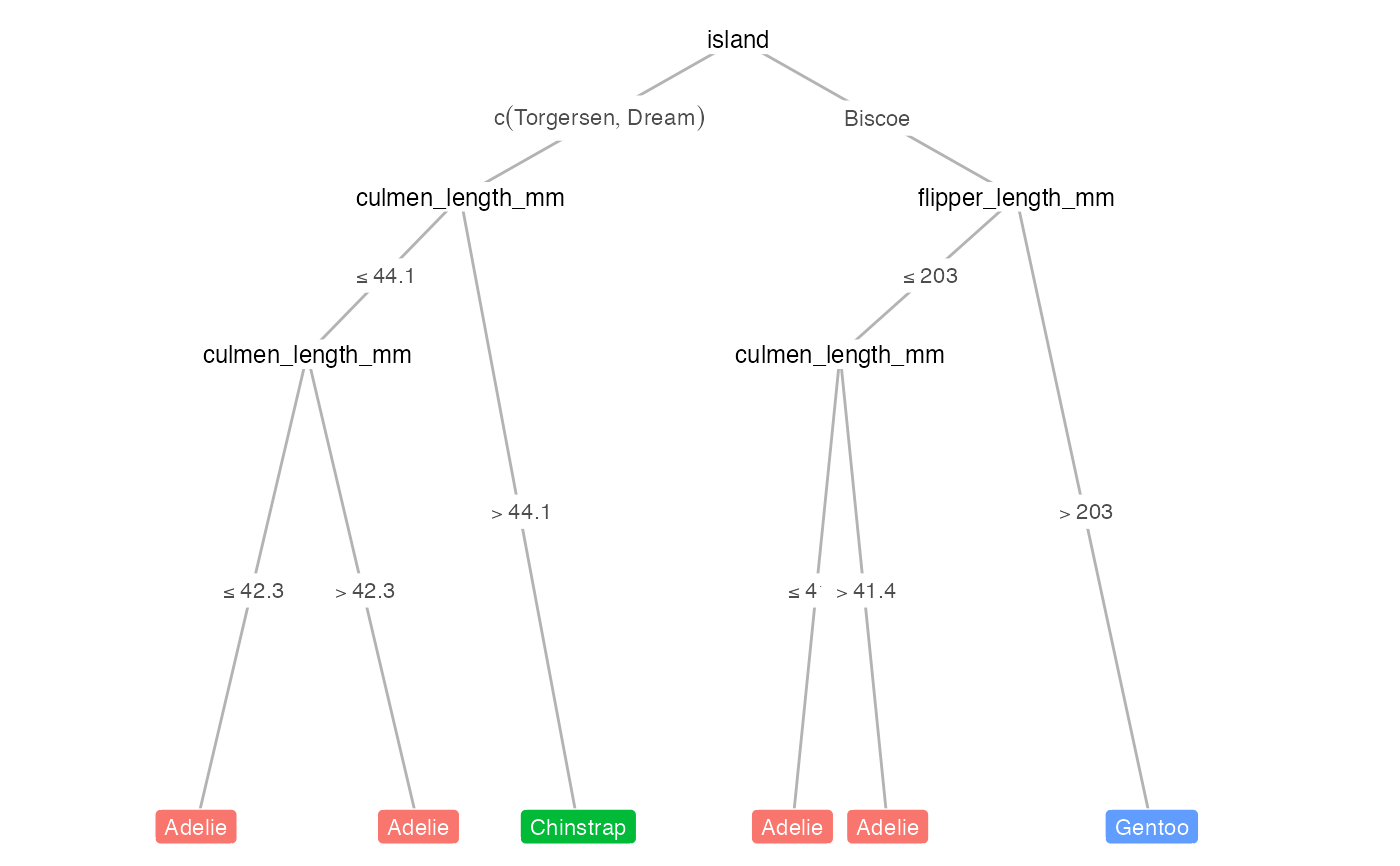Draws the conditional decision tree output from partykit::ctree(), utilizing ggparty geoms: geom_edge, geom_edge_label, geom_node_label.

draw_tree(
dat,
fit,
term_dat,
layout,
target_cols = NULL,
title = NULL,
tree_space_top = 0.05,
tree_space_bottom = 0.05,
print_eval = FALSE,
metrics = NULL,
x_eval = 0,
y_eval = 0.9,
par_node_vars = list(label.size = 0, label.padding = unit(0.15, "lines"), line_list =
list(aes(label = splitvar)), line_gpar = list(list(size = 9)), ids = "inner"),
terminal_vars = list(label.padding = unit(0.25, "lines"), size = 3, col = "white"),
edge_vars = list(color = "grey70", size = 0.5),
edge_text_vars = list(color = "grey30", size = 3, mapping = aes(label =
paste(breaks_label, "*NA")))
)

## Arguments

dat

Dataframe with samples from original dataset ordered according to the clustering within each leaf node.

fit

party object, e.g., as output from partykit::ctree()

term_dat

Dataframe for terminal nodes, must include these columns: id, x, y and y_hat.

layout

Dataframe of layout of all nodes, must include these columns: id, x, y and y_hat.

target_cols

Character vectors representing the hex values of different level colors for targets, defaults to viridis option B.

title

Character string for plot title.

tree_space_top

Numeric value to pass to expand for top margin of tree.

tree_space_bottom

Numeric value to pass to expand for bottom margin of tree.

print_eval

Logical. If TRUE, print evaluation of the tree performance.

metrics

A set of metric functions to evaluate decision tree, defaults to common metrics for classification/regression problems. Can be defined with yardstick::metric_set.

x_eval

Numeric value indicating x position to print performance statistics.

y_eval

Numeric value indicating y position to print performance statistics.

Character string indicating the type of problem, either 'classification' (categorical outcome) or 'regression' (continuous outcome).

par_node_vars

Named list containing arguments to be passed to the geom_node_label() call for non-terminal nodes.

terminal_vars

Named list containing arguments to be passed to the geom_node_label() call for terminal nodes.

edge_vars

Named list containing arguments to be passed to the geom_edge() call for tree edges.

edge_text_vars

Named list containing arguments to be passed to the geom_edge_label() call for tree edge annotations.

## Value

A ggplot2 grob object of the decision tree.

## Examples

x <- compute_tree(penguins, target_lab = 'species')
draw_tree(x$dat, x$fit, x$term_dat, x$layout)